The molecules of orthosilicic acid may lose one molecule of water, the remaining atom of oxygen of the two hydroxl groups serving to unite the two molecules together, and a similar loss of water may be repeated twice, thus:-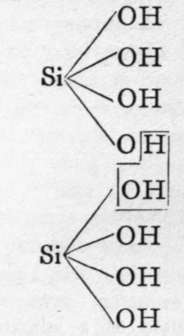Two molecules of orthosilicic acid.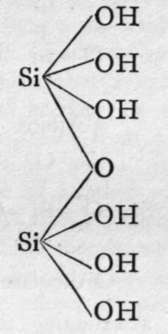Disilicic acid.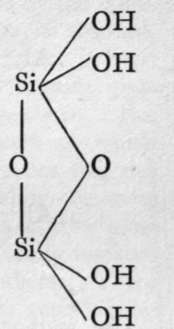1st anhydride.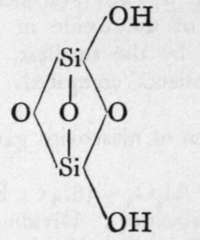2nd Anhydride.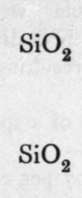Silica.

The final loss of water yields anhydrous silica. These acids are not known as such ; but certain minerals may be regarded as their salts. It is probable that okenite is a disilicate, thus CaH4Si2O7 ; and also petalite, a derivative of its second anhydride, AlLi(Si2O5)2. Similarly, three molecules of orthosilicic acid, by losing two molecules of water, may unite to form trisilicic acid; and it again by successive loss of one, two, and three molecules of water may yield a first, a second, and a third anhydride. The well-known felspars albite and orthoclase are salts of the third anhydride of trisilicic acid, thus :-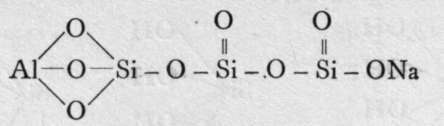Albite.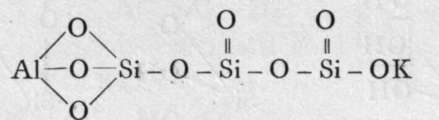Orthoclase.

The method of ascertaining the formula of a silicate requires notice. In order to determine the relative number of molecules of silica, SiO2, and of the oxides of the various metals in combination with it, each percentage is divided by the molecular weight of the oxide in question ; the quotients are then divided by the smallest, and the ratio between the resulting quotients compared. To take an instance:-

An analysis of a specimen of muscovite gave the following numbers :-

SiO2= 45.07 per cent.; A12O3 = 38.41 ; K2O = 12.10; H2O = 4«42; together = 100.00. Dividing by 60.4; by 102.3 » 94.3 ; by 18.0, the quotients 0.746, 0.375, 0.128, 0.245 are obtained. Again dividing the quotients by 0.128, the smallest of these quotients, the ratios are: 6, 3, 1, 2, nearly. Therefore the formula is 6SiO2, 3A12O3, K2O, 2H2O, or, adding the various constituents together and dividing by 2, Si3O12Al3KH2. The group Si3O12 is 3 x SiO4; the compound is, therefore, an ortho-silicate ; and three atoms of triad aluminium, one of monad potassium, and two of monad hydrogen are equivalent to the twelve atoms of hydrogen of the three molecules of orthosilicic acid. It sometimes happens, however, that one metal, such as magnesium, may replace more or less of another, such as calcium and iron. In that case, the quotients obtained on dividing the percentages by the molecular weights are added before the final ratio is obtained. The products of Nature's laboratory are seldom, if ever, pure ; and it rarely happens that a natural mineral gives results so easily interpreted as the case given above. For the mineral no doubt separates from a matrix in which many compounds are present; and so it happens that one metal may take the place of another possessing the same valency, and capable of forming compounds of the same crystalline form. The alkali metals are mutually replaceable ; also the triads Al, Fe, Mn, Cr, and others. There are even instances where silicon is partially replaced by titanium; hence the interpretation of the results of analyses presents a problem of no small difficulty. The work of F. W. Clarke, of the U.S. Geological Survey, has contributed not a little to a solution of this problem.